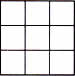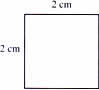Tuesday , August 20 2019
Home / 5th Class / NCERT 5th Class (CBSE) Mathematics: Area And Volume# NCERT 5th Class (CBSE) Mathematics: Area And Volume

### A. Area of

Mrs Rao has recently put new tiles in her kitchen. One way to find the are covered by the tiles would be to count them. The other way would be to use multiplication.There are 10 tiles in a row. There are 3 rows of tiles

10 × 3 = 30 tiles

Area = 30 sq. units

This is the same as multiplying the length and breadth of the rectangle.

10       ×          3      =     30 sq. units

Length × breadth = Area of a rectangle ← Formula for areas of a rectengle

(a) Length = 20 cm, Breadth = 0.1 m, Area = ?

0.1 m = 10 cm

20 cm × 10 cm = 200 sq. cm

Area = 200 cm2

(b) Area of a rectangle = 72 m2

Length = 12 m

Breadth = ?

Breadth = area/Length

72/12 = 6

breadth = 6

### B. Area of a Square

A square is a rectangle with all sides equal.

So, the area of a square = side × Side ← Formula for areas of a square

(a)Side of the square = 3 units

Area = 3 × 3 = 9 sq. units

(b)Side of the square = 2 cm

Area =2 × 2 = 4 cm2

## 8th Mathematics Summative Assessment-II (2016-17)

8th Mathematics Summative Assessment-II (2016-17) School Name: Venkateshwar Global School, Sector 13, Rohini, Delhi 110085 India Time: 3 hour …